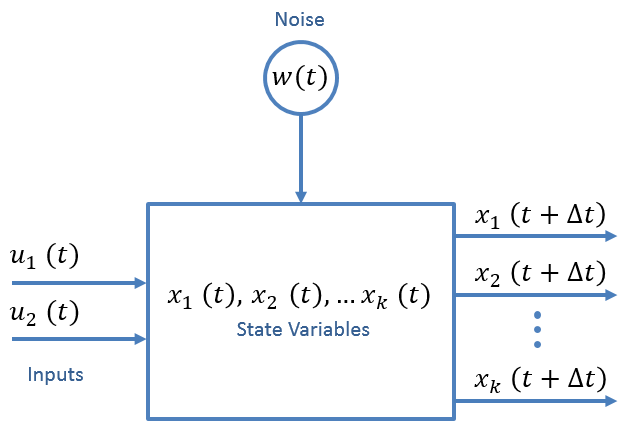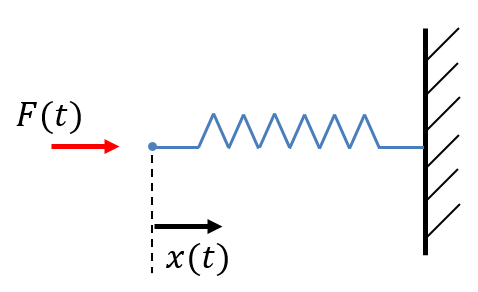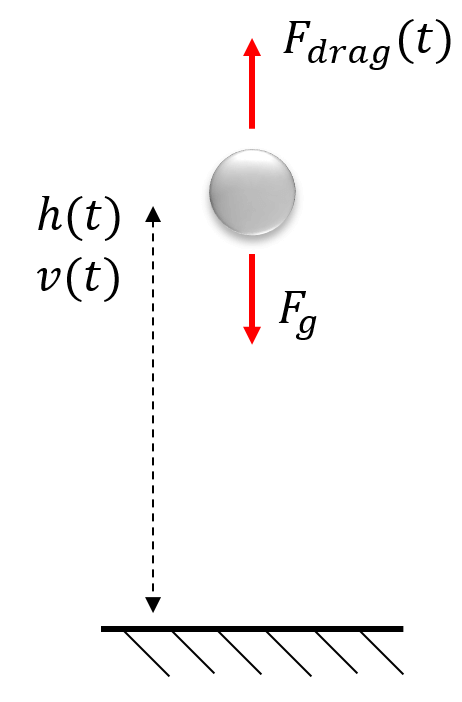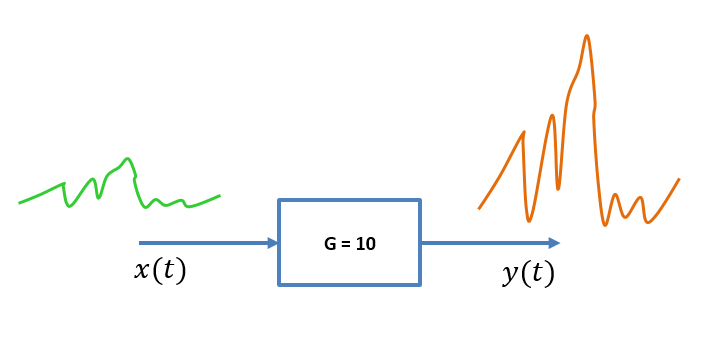﻿ State Extrapolation Equation

# State Extrapolation Equation

The first Kalman Filter equation that I would like to describe is the state extrapolation equation.

Using the state extrapolation equation, we can predict the next system state based on the knowledge of the current state. It extrapolates the state vector from the present (time step $$n$$ ) to the future (time step $$n + 1$$ ).

The state extrapolation equation describes the model of the dynamic system. In the literature, it is also called:

• Predictor Equation
• Transition Equation
• Prediction Equation
• Dynamic Model
• State Space Model

The general form of the state extrapolation equation in a matrix notation is:

$\boldsymbol{\hat{x}}_{n+1,n} = \boldsymbol{F\hat{x}}_{n,n} + \boldsymbol{Gu}_{n} + \boldsymbol{w}_{n}$
Where:
 $$\boldsymbol{\hat{x}}_{n+1,n}$$ is a predicted system state vector at time step $$n + 1$$ $$\boldsymbol{\hat{x}}_{n,n}$$ is an estimated system state vector at time step $$n$$ $$\boldsymbol{u}_{n}$$ is a control variable or input variable - a measurable (deterministic) input to the system $$\boldsymbol{w}_{n}$$ is a process noise or disturbance - an unmeasurable input that affects the state $$\boldsymbol{F}$$ is a state transition matrix $$\boldsymbol{G}$$ is a control matrix or input transition matrix (mapping control to state variables)
Note: In the literature, the state transition matrix $$F$$ is sometimes denoted by a Greek letter $$\Phi$$.

The following figure provides a schematic description of the state extrapolation equation.The state variables may represent attributes of the system that we wish to know.

For example, a moving vehicle has three attributes: position, velocity, and acceleration.

You might ask yourself, which attributes are the state variables, and which attributes are the input to the system?

• Moving mechanical systems have attributes such as position, velocity, acceleration, and drag.
• A force that acts on a system should be considered an external forcing function, i.e., an input to the system that controls the state vector (position and velocity in the constant acceleration case).
• Newton's second law tells us that $$F = ma$$. Thus we can consider acceleration as an external input to the system.
• The position and the velocity are the primary state variables of interest.

For instance, in a spring system, the force applied to the spring $$F(t)$$ is an input $$u(t)$$, while the spring displacement $$x(t)$$ is the system state.For a falling object, the inputs are the gravitational force $$F_{g}$$ and the drag force $$F_{drag}(t)$$, while the object height $$h(t)$$ and velocity $$v(t)$$ are the system states.Note: The process noise $$w_{n}$$ does not typically appear directly in the equations of interest. Instead, this term is used to model the uncertainty in the Covariance Extrapolation Equation.

Let's take a look at several examples of the state extrapolation equation.

## Example - airplane - no control input

In this example, we define the State Extrapolation Equation for an airplane, assuming a constant acceleration model.

In this example, there is no control input.

We will assume no control input. We will see the control input in the next example.

$\boldsymbol{u}_{n} = 0$

Consider an airplane moving in three-dimensional space with constant acceleration. The state vector $$\boldsymbol{\hat{x}_{n}}$$ that describes the estimated airplane position, velocity, and acceleration in a cartesian coordinate system $$\left( x,y,z \right)$$ is:

$\boldsymbol{\hat{x}}_{n}= \left[ \begin{matrix} \hat{x}_{n}\\ \hat{y}_{n}\\ \hat{z}_{n}\\ \hat{\dot{x}}_{n}\\ \hat{\dot{y}}_{n}\\ \hat{\dot{z}}_{n}\\ \hat{\ddot{x}}_{n}\\ \hat{\ddot{y}}_{n}\\ \hat{\ddot{z}}_{n}\\ \end{matrix} \right]$

Note: Don't confuse the estimated state vector $$\boldsymbol{\hat{x}_{n}}$$ (bold-face font) and the estimated airplane position at $$x$$ - axis denoted by $$\hat{x}_{n}$$ (normal-face font). Both variables are denoted by the same letter in the literature, and I am trying to be consistent.

The state transition matrix $$\boldsymbol{F}$$ is:

$\boldsymbol{F}= \left[ \begin{matrix} 1 & 0 & 0 & \Delta t & 0 & 0 & 0.5\Delta t^{2} & 0 & 0 \\ 0 & 1 & 0 & 0 & \Delta t & 0 & 0 & 0.5\Delta t^{2} & 0 \\ 0 & 0 & 1 & 0 & 0 & \Delta t & 0 & 0 & 0.5\Delta t^{2}\\ 0 & 0 & 0 & 1 & 0 & 0 & \Delta t & 0 & 0 \\ 0 & 0 & 0 & 0 & 1 & 0 & 0 & \Delta t & 0 \\ 0 & 0 & 0 & 0 & 0 & 1 & 0 & 0 & \Delta t\\ 0 & 0 & 0 & 0 & 0 & 0 & 1 & 0 & 0 \\ 0 & 0 & 0 & 0 & 0 & 0 & 0 & 1 & 0 \\ 0 & 0 & 0 & 0 & 0 & 0 & 0 & 0 & 1\\ \end{matrix} \right]$

The state extrapolation equation is:

$\boldsymbol{\hat{x}}_{n+1,n} = \boldsymbol{F\hat{x}}_{n}$

$\left[ \begin{matrix} \hat{x}_{n+1,n}\\ \hat{y}_{n+1,n}\\ \hat{z}_{n+1,n}\\ \hat{\dot{x}}_{n+1,n}\\ \hat{\dot{y}}_{n+1,n}\\ \hat{\dot{z}}_{n+1,n}\\ \hat{\ddot{x}}_{n+1,n}\\ \hat{\ddot{y}}_{n+1,n}\\ \hat{\ddot{z}}_{n+1,n}\\ \end{matrix} \right] = \left[ \begin{matrix} 1 & 0 & 0 & \Delta t & 0 & 0 & 0.5\Delta t^{2} & 0 & 0 \\ 0 & 1 & 0 & 0 & \Delta t & 0 & 0 & 0.5\Delta t^{2} & 0 \\ 0 & 0 & 1 & 0 & 0 & \Delta t & 0 & 0 & 0.5\Delta t^{2}\\ 0 & 0 & 0 & 1 & 0 & 0 & \Delta t & 0 & 0 \\ 0 & 0 & 0 & 0 & 1 & 0 & 0 & \Delta t & 0 \\ 0 & 0 & 0 & 0 & 0 & 1 & 0 & 0 & \Delta t\\ 0 & 0 & 0 & 0 & 0 & 0 & 1 & 0 & 0 \\ 0 & 0 & 0 & 0 & 0 & 0 & 0 & 1 & 0 \\ 0 & 0 & 0 & 0 & 0 & 0 & 0 & 0 & 1\\ \end{matrix} \right] \left[ \begin{matrix} \hat{x}_{n,n}\\ \hat{y}_{n,n}\\ \hat{z}_{n,n}\\ \hat{\dot{x}}_{n,n}\\ \hat{\dot{y}}_{n,n}\\ \hat{\dot{z}}_{n,n}\\ \hat{\ddot{x}}_{n,n}\\ \hat{\ddot{y}}_{n,n}\\ \hat{\ddot{z}}_{n,n}\\ \end{matrix} \right]$

The matrix multiplication results:

$\begin{cases} \hat{x}_{n+1,n} = \hat{x}_{n,n} + \hat{\dot{x}}_{n,n} \Delta t+ \frac{1}{2}\hat{\ddot{x}}_{n,n} \Delta t^{2}\\ \hat{y}_{n+1,n} = \hat{y}_{n,n} + \hat{\dot{y}}_{n,n} \Delta t+ \frac{1}{2}\hat{\ddot{y}}_{n,n} \Delta t^{2}\\ \hat{z}_{n+1,n} = \hat{z}_{n,n} + \hat{\dot{z}}_{n,n} \Delta t+ \frac{1}{2}\hat{\ddot{z}}_{n,n} \Delta t^{2}\\ \hat{\dot{x}}_{n+1,n} = \hat{\dot{x}}_{n,n} + \hat{\ddot{x}}_{n,n} \Delta t\\ \hat{\dot{y}}_{n+1,n} = \hat{\dot{y}}_{n,n} + \hat{\ddot{y}}_{n,n} \Delta t\\ \hat{\dot{z}}_{n+1,n} = \hat{\dot{z}}_{n,n} + \hat{\ddot{z}}_{n,n} \Delta t\\ \hat{\ddot{x}}_{n+1,n} = \hat{\ddot{x}}_{n,n}\\ \hat{\ddot{y}}_{n+1,n} = \hat{\ddot{y}}_{n,n}\\ \hat{\ddot{z}}_{n+1,n} = \hat{\ddot{z}}_{n,n}\\ \end{cases}$

## Example - airplane with control input

This example is similar to the previous example, but now we have a sensor connected to the pilot's controls, so we have additional information about the airplane acceleration based on the pilot's commands.

The state vector $$\boldsymbol{\hat{x}_{n}}$$ that describes the estimated airplane position and velocity in a cartesian coordinate system $$\left( x,y,z \right)$$ is:

$\boldsymbol{\hat{x}}_{n}= \left[ \begin{matrix} \hat{x}_{n}\\ \hat{y}_{n}\\ \hat{z}_{n}\\ \hat{\dot{x}}_{n}\\ \hat{\dot{y}}_{n}\\ \hat{\dot{z}}_{n}\\ \end{matrix} \right]$

The control vector $$\boldsymbol{u_{n}}$$ that describes the measured airplane acceleration in a cartesian coordinate system $$\left( x,y,z \right)$$ is:

$\boldsymbol{u}_{n}= \left[ \begin{matrix} \ddot{x}_{n}\\ \ddot{y}_{n}\\ \ddot{z}_{n}\\ \end{matrix} \right]$

The state transition matrix $$\boldsymbol{F}$$ is:

$\boldsymbol{F}= \left[ \begin{matrix} 1 & 0 & 0 & \Delta t & 0 & 0\\ 0 & 1 & 0 & 0 & \Delta t & 0\\ 0 & 0 & 1 & 0 & 0 & \Delta t\\ 0 & 0 & 0 & 1 & 0 & 0\\ 0 & 0 & 0 & 0 & 1 & 0\\ 0 & 0 & 0 & 0 & 0 & 1\\ \end{matrix} \right]$

The control matrix $$\boldsymbol{G}$$ is:

$\boldsymbol{G}= \left[ \begin{matrix} 0.5\Delta t^{2} & 0 & 0 \\ 0 & 0.5\Delta t^{2} & 0 \\ 0 & 0 & 0.5\Delta t^{2} \\ \Delta t & 0 & 0 \\ 0 & \Delta t & 0 \\ 0 & 0 & \Delta t \\ \end{matrix} \right]$

The state extrapolation equation is:

$\boldsymbol{\hat{x}}_{n+1,n} = \boldsymbol{F\hat{x}}_{n,n} + \boldsymbol{Gu}_{n,n}$

$\left[ \begin{matrix} \hat{x}_{n+1,n}\\ \hat{y}_{n+1,n}\\ \hat{z}_{n+1,n}\\ \hat{\dot{x}}_{n+1,n}\\ \hat{\dot{y}}_{n+1,n}\\ \hat{\dot{z}}_{n+1,n}\\ \end{matrix} \right] = \left[ \begin{matrix} 1 & 0 & 0 & \Delta t & 0 & 0\\ 0 & 1 & 0 & 0 & \Delta t & 0\\ 0 & 0 & 1 & 0 & 0 & \Delta t\\ 0 & 0 & 0 & 1 & 0 & 0\\ 0 & 0 & 0 & 0 & 1 & 0\\ 0 & 0 & 0 & 0 & 0 & 1\\ \end{matrix} \right] \left[ \begin{matrix} \hat{x}_{n,n}\\ \hat{y}_{n,n}\\ \hat{z}_{n,n}\\ \hat{\dot{x}}_{n,n}\\ \hat{\dot{y}}_{n,n}\\ \hat{\dot{z}}_{n,n}\\ \end{matrix} \right] + \left[ \begin{matrix} 0.5\Delta t^{2} & 0 & 0 \\ 0 & 0.5\Delta t^{2} & 0 \\ 0 & 0 & 0.5\Delta t^{2} \\ \Delta t & 0 & 0 \\ 0 & \Delta t & 0 \\ 0 & 0 & \Delta t \\ \end{matrix} \right] \left[ \begin{matrix} \ddot{x}_{n}\\ \ddot{y}_{n}\\ \ddot{z}_{n}\\ \end{matrix} \right]$

## Example – falling object

Consider a free-falling object. The state vector includes the altitude $$h$$ and the object's velocity $$\dot{h}$$:

$\boldsymbol{\hat{x}}_{n}= \left[ \begin{matrix} \hat{h}_{n}\\ \hat{\dot{h}}_{n}\\ \end{matrix} \right]$

The state transition matrix $$\boldsymbol{F}$$ is:

$\boldsymbol{F}= \left[ \begin{matrix} 1 & \Delta t \\ 0 & 1 \\ \end{matrix} \right]$

The control matrix $$\boldsymbol{G}$$ is:

$\boldsymbol{G}= \left[ \begin{matrix} 0.5\Delta t^{2} \\ \Delta t \\ \end{matrix} \right]$

The input variable $$\boldsymbol{u}_{n}$$ is:

$\boldsymbol{u}_{n}= \left[ \begin{matrix} g \end{matrix} \right]$

Where $$g$$ is the gravitational acceleration.

We don't have a sensor that measures acceleration, but we know that for a falling object, acceleration equals $$g$$.

The state extrapolation equation looks as follows:

$\left[ \begin{matrix} \hat{h}_{n+1,n}\\ \hat{\dot{h}}_{n+1,n}\\ \end{matrix} \right] = \left[ \begin{matrix} 1 & \Delta t \\ 0 & 1 \\ \end{matrix} \right] \left[ \begin{matrix} \hat{h}_{n,n}\\ \hat{\dot{h}}_{n,n}\\ \end{matrix} \right] + \left[ \begin{matrix} 0.5\Delta t^{2} \\ \Delta t \\ \end{matrix} \right] \left[ \begin{matrix} g \end{matrix} \right]$

The matrix multiplication results in the following:

$\begin{cases} \hat{h}_{n+1,n} = \hat{h}_{n,n} + \hat{\dot{h}}_{n,n} \Delta t + 0.5 \Delta t^{2} g\\ \hat{\dot{h}}_{n+1,n} = \hat{\dot{h}}_{n,n} + \Delta t g\\ \end{cases}$

## State extrapolation equation dimensions

The following table specifies the matrix dimensions of the state extrapolation equation variables:

Variable Description Dimension
$$\boldsymbol{x}$$ state vector $$n_{x} \times 1$$
$$\boldsymbol{F}$$ state transition matrix $$n_{x} \times n_{x}$$
$$\boldsymbol{u}$$ input variable $$n_{u} \times 1$$
$$\boldsymbol{G}$$ control matrix $$n_{x} \times n_{u}$$
$$\boldsymbol{w}$$ process noise vector $$n_{x} \times 1$$

## Linear time-invariant systems

The Linear Kalman Filter assumes the LTI (Linear Time-Invariant) system model.

So, what is "linear," and what is "time-invariant"?

Linear systems are described by systems of equations in which the variables are never multiplied with each other but only with constants and then summed up. Linear systems are used to describe both static and dynamic relationships between variables.

A linear system is a system whose output function $$y(t)$$ satisfies the following equation:

$y(t) = \mathcal{F} \left( a \times g \left( t \right) +b \times h \left( t \right) \right) = a \times \mathcal{F} \left( g \left( t \right) \right) + b \times \mathcal{F} \left( h \left( t \right) \right)$

Where:

$$a$$ and $$b$$ are constant real numbers

$$g$$ and $$h$$ are any arbitrary functions of an independent variable $$t$$

A linear system follows two basic rules:

1. You can "factor out" constant multiplicative scale factors (the $$a$$ and $$b$$ above).
2. The system's response to a sum of inputs is the sum of the responses to each input separately.

A time-invariant system has a system function that is not a direct function of time.

Let's take an amplifier with gain $$G = 10$$ as an example.This system is time-invariant. Although the system's output changes with time, the system function is not time-dependent.

A time-invariant system is one where a time delay (or shift) in the input sequence causes an equivalent time delay in the system's output sequence.